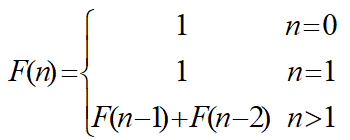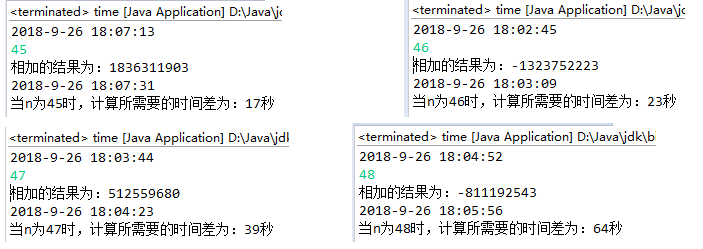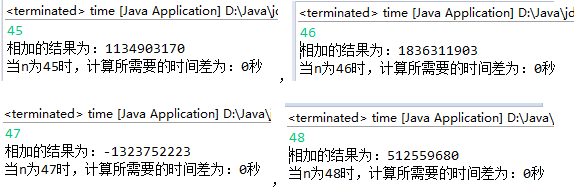• 主要为大家详细介绍了Java递归实现斐波那契数列，具有一定的参考价值，感兴趣的小伙伴们可以参考一下
• Fibonacci数列递归的实现 先来一个fibonacci数列的定义： Fibonacci数列指的是这样一个数列：1、1、2、3、5、8、13、21、34、……在数学上，斐波那契数列以如下被以递推的方法定义：F(1)=1，F(2)=1, F(n)=F(n - 1)...
 Fibonacci数列递归的实现
先来一个fibonacci数列的定义： Fibonacci数列指的是这样一个数列：1、1、2、3、5、8、13、21、34、……在数学上，斐波那契数列以如下被以递推的方法定义：F(1)=1，F(2)=1, F(n)=F(n - 1)+F(n - 2)（n ≥ 3，n ∈ N* 。 Fibonacci数列在程序中的实现还是很容易，他是一个典型的可以用递归现实的算法。我们先来一个普通的递归写法：
int fibo(int n)
{
if(n == 1 || n == 2)
return 1;
return fibo(n-1)+fibo(n-2);

}
int main()
{
int n,result;
printf("请输入：");
scanf("%d",&n);
result = fibo(n);
printf("%d\n",result);
}


递归代码简洁，但是如果不做一定的优化，很容易出现栈溢出。以上的实现就会非常耗费内存，因为当n>2时，fibo函数需要调用自身n-2次才开始有返回值，然后开逐个返回原函数并开始计算。如果要求的n值非常大的话，可能需要同时保存成千上百个调用记录，很容易发生"栈溢出"错误（stack overflow）。
但是我们可以对以上实现做一个小优化-尾递归 尾递归是指，在函数返回的时候，调用自身本身，并且，return语句不能包含表达式。这样，编译器或者解释器就可以把尾递归做优化，使递归本身无论调用多少次，都只占用一个栈帧，不会出现栈溢出的情况。 一般来说，由于只存在一个调用记录，所以永远不会发生"栈溢出"错误。 优化后的递归函数：
int fibo(int n,int i,int j)
{
if(n ==1 || n ==2)
return j;
return fibo(n-1,j,j+i);
}

int main()
{
int n,result;
printf("请输入：");
scanf("%d",&n);
result = fibo(n,1,1);
printf("%d\n",result);
}


这样一来，当fibo函数调用自身，就开始有return值，在递归结束后不用再把return值逐个返回原函数。尾递归的实现方式，编译器可以帮我们节省大量的内存消耗，妈妈再也不用我的栈溢出了！
展开全文• 斐波那契数列Fibonacci sequence），又称黄金分割数列、因数学家莱昂纳多·斐波那契（Leonardoda Fibonacci）以兔子繁殖为例子而引入，故又称为“兔子数列”，指的是这样一个数列： 0、1、1、2、3、5、8、13、21、...
斐波那契数列
斐波那契数列（Fibonacci sequence），又称黄金分割数列、因数学家莱昂纳多·斐波那契（Leonardoda Fibonacci）以兔子繁殖为例子而引入，故又称为“兔子数列”，指的是这样一个数列： 0、1、1、2、3、5、8、13、21、34、…… 在数学上，斐波那契数列以如下被以递推的方法定义： F(0)=0， F(1)=1, F(n)=F(n - 1)+F(n - 2)（n ≥ 3，n ∈ N*） 在现代物理、准晶体结构、化学等领域，斐波纳契数列都有直接的应用，为此，美国数学会从 1963 年起出版了以《斐波纳契数列季刊》为名的一份数学杂志，用于专门刊载这方面的研究成果。
用循环法：
#define _CRT_SECURE_NO_WARNINGS 1
//构建斐波那契数列
//{0,1,1,2,3,5,8,13,...}即：F(n=F(n-1)+F(n-2）
//循环法
#include<stdio.h>
int main()
{
int n = 0;
int a = 0;
int c = 0;
printf("请输入你要输出第几个斐波那契数(大于0)：\n");
scanf("%d", &n);
if (n < 0)
{
printf("请输入一个大于1的数\n");
}
else if (n==1)
{
a = 0;
printf("斐波那契数为：%d\n", a);
}
else if (n == 2)
{
a = 1;
printf("斐波那契数为：%d\n", a);
}
else
{
int a = 0;
int b = 1;

while (n > 2)
{
c = a + b;
a = b;
b = c;
n--;
}
}
printf("斐波那契数为：%d\n", c);
return 0;
}用函数的方法：
非递归方式
//非递归的方法求斐波那契数列
#include<stdio.h>
int Fib(int x)
{
if (x == 1)//当x等于1时候，直接返回0
return 0;
else if (x == 2)//当x等于2时候，直接返回1
return 1;
else
{
int first = 0;//定义第1个斐波那契数为0
int second = 1;//定义第2个斐波那契数为0
int ret = 0;//定义一个整形接收值
while (x>2)//当x大于2的时候进入循环
{
ret = first + second;
first= second;
second = ret;
x--;
}
return ret;//返回ret的值
}
}
int main()
{
int n = 0;

printf("请输入你要输出第几个斐波那契数(大于0)：\n");
scanf("%d", &n);
if (n > 0)
{
printf("第%d个斐波那契数为：%d\n",n, Fib(n));
}
else
{
printf("请输入一个大于1的数\n");
}
return 0;
}递归方式
//递归的方法求斐波那契数列
#include<stdio.h>
int Fib(int x)
{
if (x == 1)
{
return 0;//第一个斐波那契数为0
}

else if (x == 2)
{
return 1;//第二个斐波那契数为1
}
else
{
return Fib(x - 1) + Fib(x - 2);//从第三个数开始就回去找Fib（2）和Fib（1）
//不断回去找直到找到为止
}
}
int main()
{
int n = 0;
printf("请输入你要输出第几个斐波那契数(大于0)：\n");
scanf("%d", &n);
if (n > 0)
{
printf("第%d个斐波那契数为：%d\n",n, Fib(n));
}
else
{
printf("请输入一个大于1的数\n");
}
return 0;
}求斐波那契数列前几项的和
代码1：
#include<stdio.h>
int FibSum(int x)
{
if (x == 1)//前1项的和为0
{
return 0;
}
else if (x == 2)//前2项的和为1
{
return  1;
}
else
{
int first = 0;
int second = 1;
int sum = 1;//从第3项开始计算和，没有加前两项的和，所以sum初始化为1
int ret = 0;
while (x > 2)
{
ret = first + second;
first = second;
second = ret;
sum += ret;//每次求出的斐波那契数加在sum上
x--;
}
return sum;
}
}
int main()
{
int  n = 0;
printf("请输入你要求前几项的和：\n");
scanf("%d", &n);
if (n > 0)
{
printf("前%d项斐波那契数的和为：%d\n",n,FibSum(n));
}
else
{
printf("请输入一个大于0的数\n");
}
return 0;
}代码2：
//求菲波那切数列前几项的和
//递归方法
#include<stdio.h>
int Fib(int x)
{
if (x == 1)
{
return 0;
}
else if (x == 2)
{
return 1;
}
else
{
return  Fib(x-1) + Fib(x - 2);

}

}
int main()
{
int  n = 0;
printf("请输入你要求前几项的和：\n");
scanf("%d", &n);
if (n > 0)
{
int sum = 0;
for (int i = 1; i <= n; i++)//利用for循环求和
{
sum += Fib(i);

}
printf("前%d项斐波那契数的和为：%d\n", n,sum );
}
else
{
printf("请输入一个大于0的数\n");
}
return 0;
}总结： 如果所求的斐波那契数或前n项和数字比较小的话，可能使用递归和不使用区别不大，但是当数字特别大的时候，使用递归的话，速度会非常慢，因为做了很多的重复计算（因为要不停的回去找前面的数）
展开全文c语言
• 用递归的方法求斐波那契数列 要解决这个问题需要了解斐波那契定义斐波那契数列Fibonacci sequence），又称黄金分割数列，因数学家莱昂纳多·斐波那契（Leonardoda Fibonacci）以兔子繁殖为例子而引入，故又...
用递归的方法求斐波那契数列
要解决这个问题需要了解斐波那契的定义： 斐波那契数列（Fibonacci sequence），又称黄金分割数列，因数学家莱昂纳多·斐波那契（Leonardoda Fibonacci）以兔子繁殖为例子而引入，故又称为“兔子数列”，指的是这样一个数列：0、1、1、2、3、5、8、13、21、34、……在数学上，斐波那契数列以如下被以递推的方法定义：F(0)=0，F(1)=1, F(n)=F(n - 1)+F(n - 2)（n ≥ 2，n ∈ N*） 由上方可知道递推公式为：F(n)=F(n - 1)+F(n - 2) 那么不用递推的方法可以写出：
n=20
a,b=0,1
for i in range(1,n+1):
a,b=b,a=b
print(a)

这里举个求第二十项的例子。 把F(n)=F(n - 1)+F(n - 2)，用递归函数翻译这个式子。 首先： 由F(2)=F(1)+F(0)=1，可以知道F(1)=1，F(0)=0， 那么可以定义函数：
def f(n):
if n==0:
return 0
elif n==1:
return 1
else:return f(n-1)+f(n-2)
print(f(n))#打印返回值，n自定义

展开全文• 【问题描述】编写函数f，功能是用递归的方法求斐波那契数列的第n项，函数原型为 int f(int n),在主函数中输入一个正整数n，调用函数f求出斐波那契数列的第n项，并在主函数中输出。 斐波那契数列：1,1,2,3,5,8,13,...
•  //使用递归计算指定位数的斐波那契数列值 //Fn=F(n-1)+F(n-2) public static int GetFibonacciNumber(int index) { if(index<0||index==0)throw new Exception(“参数不能小于或等于0”); if(index<=2)...
• 递归法计算Fibonacci数列： 它可以递归定义为： 第n个Fibonacci数列递归地计算如下： int fibonacci(int n)  {  if (n &...以下这个源代码可以计算出递归法实现Fibonacci数列时...
递归法计算Fibonacci数列：
它可以递归地定义为：第n个Fibonacci数列可递归地计算如下：
int fibonacci(int n)
{
if (n <= 1) return 1;
return fibonacci(n-1)+fibonacci(n-2);
}
以下这个源代码可以计算出递归法实现Fibonacci数列时，n为45、46、47、48时所需的时间。
import java.text.DateFormat;
import java.util.Date;
import java.util.Scanner;
public class time {
public static void main(String[] args) {

Date date=new Date();
DateFormat df=DateFormat.getDateTimeInstance();

System.out.println(df.format(date));
Scanner in=new Scanner(System.in);
int n=in.nextInt();

time a=new time();
a.Fib(n);
System.out.println("相加的结果为："+Fib(n));

Date date1=new Date();
System.out.println(df.format(date1));
long time=(date1.getTime()-date.getTime())/1000;
System.out.println("当n为"+n+"时，计算所需要的时间差为："+time+"秒");
}
public static int Fib(int n){
if(n<=1) return 1;
return Fib(n-1)+Fib(n-2);
}
}可以看到，递归法所需要的时间还是很久的，因为计算F(n)时，需首先计算F（n-1）和F(n-2)
，而在计算F（n-1）时已经算过F(n-2)了，但是递归算法看不到这点，所以产生这么多假发，所需时间也就多了，效率就下降了。
尾递归：
尾递归比递归函数效率高太多了，尾递归就是把当前的运算结果（或路径）放在参数里传给下层函数。而不是把下层函数的运算结果用来本次的计算。尾递归是极其重要的，不用尾递归，函数的堆栈耗用难以估量，需要保存很多中间函数的堆栈，而递归每一次计算出来的部分结果，在下一次循环时还需要再计算一遍。
import java.text.DateFormat;
import java.util.Date;
import java.util.Scanner;
public class time {
public static void main(String[] args) {

Date date=new Date();
DateFormat df=DateFormat.getDateTimeInstance();
Scanner in=new Scanner(System.in);
int n=in.nextInt();

time a=new time();
a.Fib(n);
System.out.println("相加的结果为："+Fib(n));
Date date1=new Date();
long time=(date1.getTime()-date.getTime())/1000;
System.out.println("当n为"+n+"时，计算所需要的时间差为："+time+"秒");
}
public static int Fib(int n){
if(n<2) return n;
return Fibo(n,1,1,3);
}
public static int Fibo(int n,int r1,int r2,int begin){
if(n==begin) return r1+r2;
return Fibo(n,r2,r1+r2,++begin);
}
}展开全文• 首先，对于斐波那契数列，我们是非常熟悉了，对斐波那契定义为如下：f(0)=0,f(1)=0,f(2)=1,……f(n)=f(n-1)+f(n-2)，其中n>1。对于这种求斐波那契数列第n项的问题，我们大多采用递归来解决。那什么是递归呢？...
• 主要内容摘自...递归一般用于解决三类问题：　(1)数据的定义是按递归定义的。（Fibonacci函数，n的阶乘） 　(2)问题解法按递归实现。（回溯） 　(3)数据的结构形式是按递归定义的。
• 斐波那契（Leonardoda Fibonacci）以兔子繁殖为例子而引入，故又称为“兔子数列”，指的是这样一个数列：0，1、1、2、3、5、8、13、21、34、……在数学上，斐波纳契数列以如下被以递归的方法定义：F(1)=1，F(2)=1, F...
• 函数递归斐波那契数列 //函数递归斐波那契数列 //编写程序，求数列1,1,2,3,5,8,13,21,…… //思路: //第一步:找出表示数列第N项的递归公式:F(N)=F(N-1)+F(N-2) //第二步:递归的结束条件，当N=1或N=2时，F(N)=1; ...c++ c语言
• 其次，定义一个feibonaqie类，构造出方法，由题目易知，当n=1和2时，数列都为1，从第三位开始满足公式，所以可以利用if-else语句来安排条件。 最后，编写一个测试类，调用feibonaqie类中的f（）方法 public ...java
• 斐波那契数列： 0，1，1，2，3，5，8，13，21 … 分析数列可以得出第n列...用递归方式（时间复杂度O(2^N)）： func fibo(n int) int { if n <= 0 { return 0 } else if n == 1 { return 1 } else { return
• 斐波那契（Leonardoda Fibonacci）以兔子繁殖为例子而引入，故又称为“兔子数列”，指的是这样一个数列：1、1、2、3、5、8、13、21、34、……在数学上，斐波纳契数列以如下被以递推的方法定义：F(1)=1，F(2)=1, F(n)...
• python中使用递归实现斐波那契数列 python中使用递归实现斐波那契数列 先来了解一下 斐波那契数列Fibonacci sequence），又称黄金分割数列、因数学家莱昂纳多·斐波那契（Leonardoda Fibonacci）以兔子繁殖为例子...python 算法
• C语言编写的斐波那契数列程序 递归 C语言初学者必会
• 递归算法Q1——斐波那契数列 /* 有一对兔子，从出生后第3个月起，每个月都生一对兔子，小兔子长到第三个月后每个月又生一对兔子。 假如兔子都不死，求第n个月兔子对数 关于斐波那契数列的兔子繁殖问题其实如下： ...
• 斐波那契（Leonardoda Fibonacci）以兔子繁殖为例子而引入，故又称为“兔子数列”，指的是这样一个数列：1、1、2、3、5、8、13、21、34、……在数学上，斐波纳契数列以如下被以递推的方法定义： F(1)=1 F(2)=1 F(n)...
• C++实现Fibonacci数列递归及非递归算法
• # include using namespace std ; int FBNQ ( int ) ; int answer = 0 ; int ...由于斐波那契数列的每一项都可以分为前两项之和，所以适合用递归作此“重复且相似的操作”
• 斐波那契数列：指的是这样一个数列 0, 1...思路：这个题我们需要用到递归的方法，首先我们需要先解决数列前两项已知的项，我们首先定义一个静态函数feibonaqie（）， 在函数当中，我们先利用一个if语句去判断前两项，如java 算法
• 编程语言中，函数Func(Type a,……)直接或间接调用函数本身，则该函数称为递归函数。递归函数不能定义为内联...求数列第n项可以用递归函数，只有当n1和n2时函数结束；其他情况一直调用他本身。 代码： #include <st
• 这就是著名的斐波那契数列，也称作兔子数列。 一、问题分析 刚开始，有1对幼兔，兔子总对数为1； 经过一个月后，幼兔长为小兔，兔子总对数为1； 经过二个月后，幼兔长大为成年兔子，并生出1对幼兔，兔子总对数...迭代算法 复杂度
• 在数学上，斐波那契数列以如下被以递推的方法定义：F(1)=1，F(2)=1, F(n)=F(n-1)+F(n-2)（n>=3，n∈N*）。分别用递归和非递归的方式，计算第n个斐波那契数。 实现代码 1、递归方法 def Fib(n): if n==1 or n==2:...python Collatz猜想 算法
• # -*- coding:utf-8 -*- #递归实现 def Fibonacci(n): if n return n return (Fibonacci(n-1) + Fibonacci(n-2))#非递归实现 class Solution: def Fibonacci(self, n): a = [0,1] i
• 斐波那契数列Fibonacci sequence），又称黄金分割数列、因数学家列昂纳多·斐波那契（Leonardoda Fibonacci）以兔子繁殖为例子而引入，故又称为“兔子数列”，指的是这样一个数列：1、1、2、3、5、8、13、21、34、...
• 斐波那契（Leonardoda Fibonacci）以兔子繁殖为例子而引入，故又称为“兔子数列”，指的是这样一个数列：1、1、2、3、5、8、13、21、34、……在数学上，斐波纳契数列以如下被以递归的方法定义：F(1)=1，F(2)=1, F(n)...
• function fn(a) {//定义函数 用递归 if (a ) {//临界值设置 return 1; } else { return fn(a - 1) + fn(a - 2);//调用自身 } } btn.onclick = function () {//点击事件 var num1 = ......